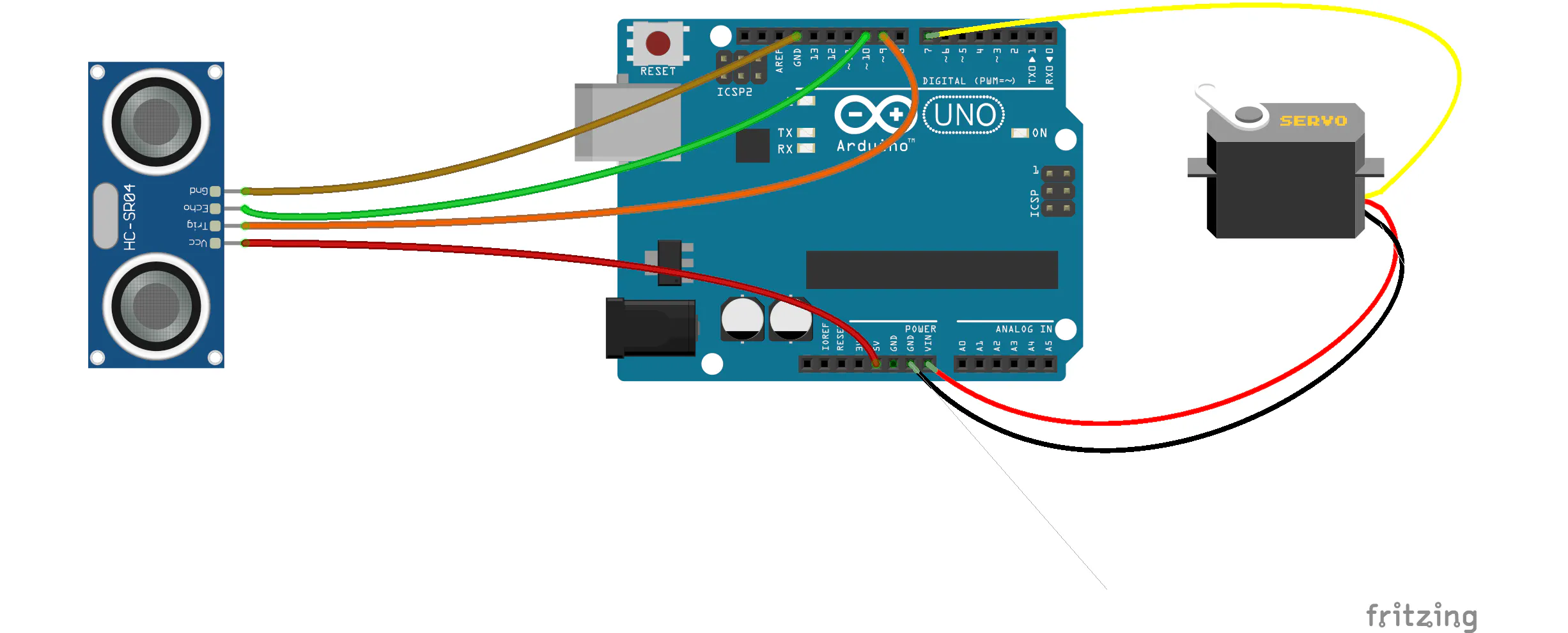# Wash-A-Lot-Bot! A DIY Hand Washing Timer 🧼

An easy way to make a hand-washing timer, using Arduino.

BeginnerFull instructions provided1 hour8,965

## Schematics

### Hand Wash Timer - Wiring Diagram## Code

Arduino
Works the best in more sinks, but may be more difficult to modify and extend.
```#include <NewPing.h> // Provides Accurate, Non-blocking sensor data from the Ultrasonic Sensor
#include <Ewma.h>    // Smoothing library - used to remove jitter from sensor data
#include <cppQueue.h> // Queue Library - used to implment mean subttraction so sensor can work in more sinks.

#include <Servo.h>

#define TRIGGER_PIN  9  // Arduino pin tied to trigger pin on the ultrasonic sensor.
#define ECHO_PIN     10  // Arduino pin tied to echo pin on the ultrasonic sensor.
#define MAX_DISTANCE 200 // Maximum distance we want to ping for (in centimeters). Maximum sensor distance is rated at 400-500cm.

#define IMPLEMENTATION FIFO //Queue implementation as First in First out
#define OVERWRITE      true //Queue will overwrite oldest value when full

int queue_length = 20;
int num_buffer_frames = 50;
int frames_since_launch = 0;
boolean isFirstLaunch = true;
int difference_threshold = 15; //the amount of deviation from the mean that will trigger the countdown.

NewPing sonar(TRIGGER_PIN, ECHO_PIN, MAX_DISTANCE);
Servo countServo;
Queue window(sizeof(float), queue_length, IMPLEMENTATION, OVERWRITE);

Ewma adcFilter1(0.05); //filter used to smooth sensor data

void setup() {

Serial.begin(115200);

//attach pin 7 to servo and sweep it to indicate that the timer is on
countServo.attach(7);
countServo.write(180);
delay(700);
countServo.write(0);
delay(700);
countServo.write(180);

clearQueue();

}

void loop() {
delay(50); // Wait 50ms between ultrasonic pings (about 20 pings/sec).

float filtered1 = adcFilter1.filter(sonar.ping_cm()); //get filtered data from the sensor
float filtered_mean_removed_value = filtered1 - meanZero(filtered1); //subtract the mean so sensor data is centered around zero.
//This allows the sensor to adjust to different environments and look for change rather than a preset threshold.

Serial.println(filtered_mean_removed_value);
if (isFirstLaunch) {
frames_since_launch++;
}
//If the sensor reads something significantly different from what it's normally seeing (the empty sink)
//we start the countdown!
if (filtered_mean_removed_value > 15 || filtered_mean_removed_value < -15) {
if (!isFirstLaunch) {
countdownServo();
}

}

if (frames_since_launch >= num_buffer_frames) {
isFirstLaunch = false;
}

}

//helper function to calculate a moving mean.
int meanZero(float smoothedVal) {

window.push(&smoothedVal); //push the most recent reading into the queue

int retval = 0;

//iterate through the queue and add values to return val
for (int i = 0; i < queue_length; i++) {
float for_mean;
window.peekIdx(&for_mean, i);
retval = retval + (int)for_mean;
}

//calculate the mean and return.
int mean = retval / queue_length;

return mean;
}

void clearQueue() {
for (int i = 0; i < queue_length; i++) {
float queue_zeroer = 0;
window.push(&queue_zeroer);
}
}

//this moves the servo 4.5 degrees every half second
//to smooth out the movemnt (vs 9 degrees every second)
//for 20 seconds.
void countdownServo() {
Serial.println("Counting down");
int halfseconds = 40;

for (int i = halfseconds; i >= 0; i--) {

countServo.write((int)(i * 4.5));
delay(500);
}

//reset the servo, clear the queue
countServo.write(180);
clearQueue();
delay(700);
frames_since_launch = 0;
isFirstLaunch = true;
}
```

### Simple Timer Code

Arduino
Easy to edit and extend, but may cause many false positives.
```#include <Servo.h>

const int trigPin = 9;
const int echoPin = 10;

float duration, distance;

//this is the threshold where the sensor will trigger the
//countdown. If you are getting extra countdowns or the
//countdown won't start, you may need to adjust this for your sink
int threshold = 200;
Servo countServo;

void setup() {

Serial.begin(9600);

//attach pin 7 to servo and sweep it to indicate that the timer is on
countServo.attach(7);
countServo.write(180);
delay(700);
countServo.write(0);
delay(700);
countServo.write(180);
}

void loop() {
digitalWrite(trigPin, LOW);
delayMicroseconds(2);
digitalWrite(trigPin, HIGH);
delayMicroseconds(10);
digitalWrite(trigPin, LOW);

duration = pulseIn(echoPin, HIGH);
distance = (duration * .0343) / 2;
Serial.println(distance);

if (distance < threshold) {
countdownServo();
}
delay(100);
}

//this moves the servo 4.5 degrees every half second
//to smooth out the movemnt (vs 9 degrees every second)
//for 20 seconds.
void countdownServo() {
Serial.println("Counting down");
int halfseconds = 40;

for (int i = halfseconds; i >= 0; i--) {

countServo.write((int)(i * 4.5));
delay(500);
}

//reset the servo, clear the queue
countServo.write(180);
delay(700);
}
```

## Credits

### Gautam Bose

1 project • 5 followers
Fiver @ Google Creative Lab Started with Potato Cannons now I'm here.

### Lucas Ochoa

1 project • 5 followers